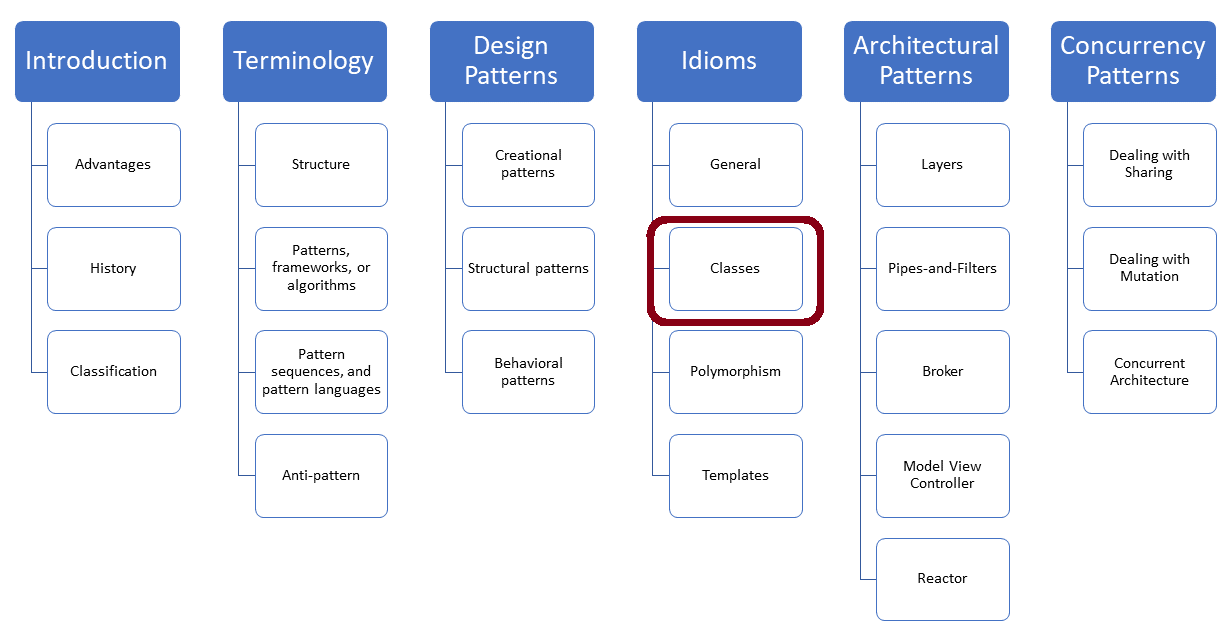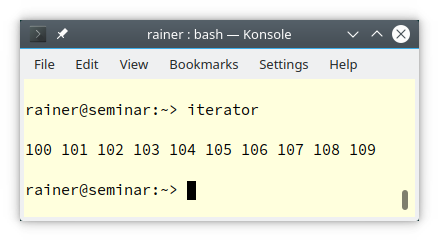# The Iterator Protocol

When you want to use a user-defined type in a range-based for-loop, your user-defined type has to implement the Iterator Protocol.Here is the question I want to answer: What interface must a user-defined type support to be usable in a range-based for-loop.

## Requirements of a Range-Based for-Loop

Let me start with a simple experiment and use ` std::array` in C++ Insights. Here is a simple example:

```// iteratorProtocol.cpp

#include <array>

int main() {

std::array<int, 5> myArr{1, 2, 3, 4, 5};
for (auto a: myArr) a;

}
```

C++ Insights creates the following code out of it:

##Modernes C++ Mentoring

Be part of my mentoring programs:

• "Fundamentals for C++ Professionals" (open)
• "Design Patterns and Architectural Patterns with C++" (open)
• "C++20: Get the Details" (reopens December 2023)
• Do you want to stay informed about my mentoring programs: Subscribe via E-Mail.

```#include <array>

int main()
{
std::array<int, 5> myArr = {{1, 2, 3, 4, 5}};
{
std::array<int, 5> & __range1 = myArr;
int * __begin1 = __range1.begin();
int * __end1 = __range1.end();
for(; __begin1 != __end1; ++__begin1) {
int a = *__begin1;
a;
}

}
return 0;
}
```

Let me write it more generally: When you use a range-based for-loop (`for(range_declaration : range_expression)`), the compiler creates the following code:

```{
auto && __range = range_expression ;
auto __begin = begin_expr;
auto __end = end_expr;
for (;__begin != __end; ++__begin) {
range_declaration = *__begin;
loop_statement
}
}
```

I marked the essential parts in red:

• `begin_expr `and `end_expr`: return an iterator object

• Iterator object
• `operator++`: incrementing the iterator
• `operator*`: dereferencing the iterator and accessing the current element
• `operator!=`: comparing the iterator with another iterator

`begin_expr` and `end_expr` call the essential `begin` and `end` on the range_expression. begin and end could either be member functions or free functions on range_expression.

Let me apply the theory and create a number generator.

## A Generator

My first implementation supports the Iterator Protocol

### The Iterator Protocol

The following class `Generator` supports the elementary Iterator Protocol.

```// iterator.cpp

#include <iostream>

class Generator {
int begin_{};
int end_{};

public:
Generator(int begin, int end) : begin_{begin}, end_{end} {}

class Iterator {
int value_{};
public:
explicit Iterator(int pos) : value_{pos} {}

int operator*() const { return value_; }           // (3)

Iterator& operator++() {                           // (4)
++value_;
return *this;
}

bool operator!=(const Iterator& other) const {      // (5)
return value_ != other.value_;
}
};

Iterator begin() const { return Iterator{begin_}; }     // (1)
Iterator end() const { return Iterator{end_}; }         // (2)
};

int main() {

std::cout << '\n';

Generator gen{100, 110};
for (auto v : gen) std::cout << v << " ";

std::cout << "\n\n";

}
```

The class `Generator` has member functions `begin` and `end, `(lines 1 and 2)` `returning iterator objects, initialized with `begin_` and` end_. begin_` and `end_` stand for the range of created numbers. Let me analyze the inner class `Iterator` which keeps track of the generated numbers:` `

• `operator*` returns the current value
• `operator++` increments the current value
• `operator!=` compares the current value with the `end_` marker.

Finally, here is the output of the program:Let me generalize the iterator returned by` begin()` and `end()` and make it a forward iterator. Afterward, the class `Generator` can be used in most of the algorithms of the Standard Template Library. To put it differently, the unordered associative containers support a forward iterator.

### A Forward Iterator

The following improved `Generator` has an inner class Iterator that is a forward iterator.

```// forwardIterator.cpp

#include <iostream>
#include <numeric>

class Generator {
int begin_{};
int end_{};

public:
Generator(int begin, int end) : begin_{begin}, end_{end} {}

class Iterator {
using iterator_category = std::forward_iterator_tag;    // (1)
using difference_type   = std::ptrdiff_t;
using value_type        = int;
using pointer           = int*;
using reference         = int&;
int value_{};
public:
explicit Iterator(int pos) : value_{pos} {}

value_type operator*() const { return value_; }
pointer operator->() { return &value_; }                // (2)

Iterator& operator++() {
++value_;
return *this;
}
Iterator operator++(int) {                              // (3)
Iterator tmp = *this;
++(*this);
return tmp;
}
// (4)
friend bool operator==(const Iterator& fir, const Iterator& sec) {
return fir.value_ == sec.value_;
}
friend bool operator!=(const Iterator& fir, const Iterator& sec) {
return fir.value_ != sec.value_;
}
};

Iterator begin() const { return Iterator{begin_}; }
Iterator end() const { return Iterator{end_}; }
};

int main() {

std::cout << '\n';

Generator gen{1, 11};
for (auto v : gen) std::cout << v << " ";                  // (5)

std::cout << "\n\n";
// (6)
std::cout << "sum:  " << std::accumulate(std::begin(gen), std::end(gen), 0);

std::cout << "\n\n";
// (7)
std::cout << "prod: " << std::accumulate(gen.begin(), gen.end(), 1,
[](int fir, int sec){ return fir * sec; });

std::cout << "\n\n";

}
```

First, `Iterator` needs several type aliases in the following member function declarations. Additionally, to the previous `Iterator` implementation in the program `iterator.cpp`, the current Iterator supports the following member functions: the arrow operator (`operator->` in line 2), the post-increment operator (`operator++(int)` in line 3), and the equal operator (`operator==` in line 4).

That was it already. Now, I can use my improved `Generator` still in a range-based for-loop (line 5), but also in the STL algorithm `std::accumulate`. Line 6 calculates the sum of all numbers from 1 to 10; line 7 does a similar job by multiplying numbers from 1 to 11. In the first case, I choose the neutral element 0 for the summation, and in the second case the neutral element 1 for the multiplication.

There is a subtle difference between the first and the second call of `std::accumulate`. The first call uses the non-member functions `std::begin` and `std::end `on the Generator: `std::accumulate(std::begin(gen), std::end(gen), 0)`, but the second call the `Generator'`s member functions `begin()` and `end()` directly which I implemented.

Finally, here is the output of the program:## What’s Next?

In my next post, I will write about the Covariant Return Type. The Covariant Return Type of a member function allows an overriding member function to return a narrower type. This is particularly useful when you implement the creational design pattern Prototype.

Thanks a lot to my Patreon Supporters: Matt Braun, Roman Postanciuc, Tobias Zindl, G Prvulovic, Reinhold Dröge, Abernitzke, Frank Grimm, Sakib, Broeserl, António Pina, Sergey Agafyin, Андрей Бурмистров, Jake, GS, Lawton Shoemake, Jozo Leko, John Breland, Venkat Nandam, Jose Francisco, Douglas Tinkham, Kuchlong Kuchlong, Robert Blanch, Truels Wissneth, Kris Kafka, Mario Luoni, Friedrich Huber, lennonli, Pramod Tikare Muralidhara, Peter Ware, Daniel Hufschläger, Alessandro Pezzato, Bob Perry, Satish Vangipuram, Andi Ireland, Richard Ohnemus, Michael Dunsky, Leo Goodstadt, John Wiederhirn, Yacob Cohen-Arazi, Florian Tischler, Robin Furness, Michael Young, Holger Detering, Bernd Mühlhaus, Matthieu Bolt, Stephen Kelley, Kyle Dean, Tusar Palauri, Dmitry Farberov, Juan Dent, George Liao, Daniel Ceperley, Jon T Hess, Stephen Totten, Wolfgang Fütterer, Matthias Grün, Phillip Diekmann, Ben Atakora, Ann Shatoff, Rob North, and Bhavith C Achar.

Thanks, in particular, to Jon Hess, Lakshman, Christian Wittenhorst, Sherhy Pyton, Dendi Suhubdy, Sudhakar Belagurusamy, Richard Sargeant, Rusty Fleming, John Nebel, Mipko, Alicja Kaminska, Slavko Radman, and David Poole.

 My special thanks to EmbarcaderoMy special thanks to PVS-StudioMy special thanks to Tipi.buildMy special thanks to Take Up Code## Seminars

I’m happy to give online seminars or face-to-face seminars worldwide. Please call me if you have any questions.

### Standard Seminars (English/German)

Here is a compilation of my standard seminars. These seminars are only meant to give you a first orientation.

• C++ – The Core Language
• C++ – The Standard Library
• C++ – Compact
• C++11 and C++14
• Concurrency with Modern C++
• Design Pattern and Architectural Pattern with C++
• Embedded Programming with Modern C++
• Generic Programming (Templates) with C++

#### New

• Clean Code with Modern C++
• C++20

### Contact Me

Modernes C++ Mentoring,Tags:
0 replies